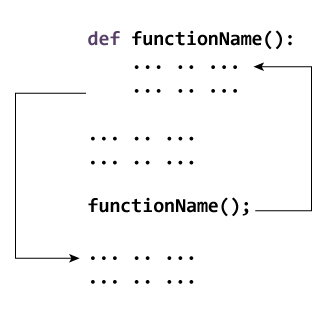# Python Functions

In this article, you'll learn about functions; what is a function, the syntax, components and types of a function. Also, you'll learn to create a function in Python.

## What is a function in Python?

In Python, function is a group of related statements that perform a specific task.

Functions help break our program into smaller and modular chunks. As our program grows larger and larger, functions make it more organized and manageable.

Furthermore, it avoids repetition and makes code reusable.

### Syntax of Function

```def function_name(parameters):
"""docstring"""
statement(s)
```

Above shown is a function definition which consists of following components.

1. Keyword `def` marks the start of function header.
2. A function name to uniquely identify it. Function naming follows the same rules of writing identifiers in Python.
3. Parameters (arguments) through which we pass values to a function. They are optional.
4. A colon (:) to mark the end of function header.
5. Optional documentation string (docstring) to describe what the function does.
6. One or more valid python statements that make up the function body. Statements must have same indentation level (usually 4 spaces).
7. An optional `return` statement to return a value from the function.

### Example of a function

```def greet(name):
"""This function greets to
the person passed in as
parameter"""
print("Hello, " + name + ". Good morning!")
```

### How to call a function in python?

Once we have defined a function, we can call it from another function, program or even the Python prompt. To call a function we simply type the function name with appropriate parameters.

```>>> greet('Paul')
Hello, Paul. Good morning!

```

Note: Try running the above code into the Python shell to see the output.

## Docstring

The first string after the function header is called the docstring and is short for documentation string. It is used to explain in brief, what a function does.

Although optional, documentation is a good programming practice. Unless you can remember what you had for dinner last week, always document your code.

In the above example, we have a docstring immediately below the function header. We generally use triple quotes so that docstring can extend up to multiple lines. This string is available to us as `__doc__` attribute of the function.

For example:

Try running the following into the Python shell to see the output.

``````>>> print(greet.__doc__)
This function greets to
the person passed into the
name parameter
```
```

## The return statement

The `return` statement is used to exit a function and go back to the place from where it was called.

### Syntax of return

```return [expression_list]
```

This statement can contain expression which gets evaluated and the value is returned. If there is no expression in the statement or the `return` statement itself is not present inside a function, then the function will return the `None` object.

For example:

``````>>> print(greet("May"))
Hello, May. Good morning!
None
```
```

Here, `None` is the returned value.

### Example of return

```def absolute_value(num):
"""This function returns the absolute
value of the entered number"""

if num >= 0:
return num
else:
return -num

# Output: 2
print(absolute_value(2))

# Output: 4
print(absolute_value(-4))
```

## How Function works in Python?## Scope and Lifetime of variables

Scope of a variable is the portion of a program where the variable is recognized. Parameters and variables defined inside a function is not visible from outside. Hence, they have a local scope.

Lifetime of a variable is the period throughout which the variable exits in the memory. The lifetime of variables inside a function is as long as the function executes.

They are destroyed once we return from the function. Hence, a function does not remember the value of a variable from its previous calls.

Here is an example to illustrate the scope of a variable inside a function.

```def my_func():
x = 10
print("Value inside function:",x)

x = 20
my_func()
print("Value outside function:",x)
```

Output

```Value inside function: 10
Value outside function: 20

```

Here, we can see that the value of x is 20 initially. Even though the function `my_func()` changed the value of x to 10, it did not effect the value outside the function.

This is because the variable x inside the function is different (local to the function) from the one outside. Although they have same names, they are two different variables with different scope.

On the other hand, variables outside of the function are visible from inside. They have a global scope.

We can read these values from inside the function but cannot change (write) them. In order to modify the value of variables outside the function, they must be declared as global variables using the keyword `global`.

## Types of Functions

Basically, we can divide functions into the following two types:

1. Built-in functions - Functions that are built into Python.
2. User-defined functions - Functions defined by the users themselves.